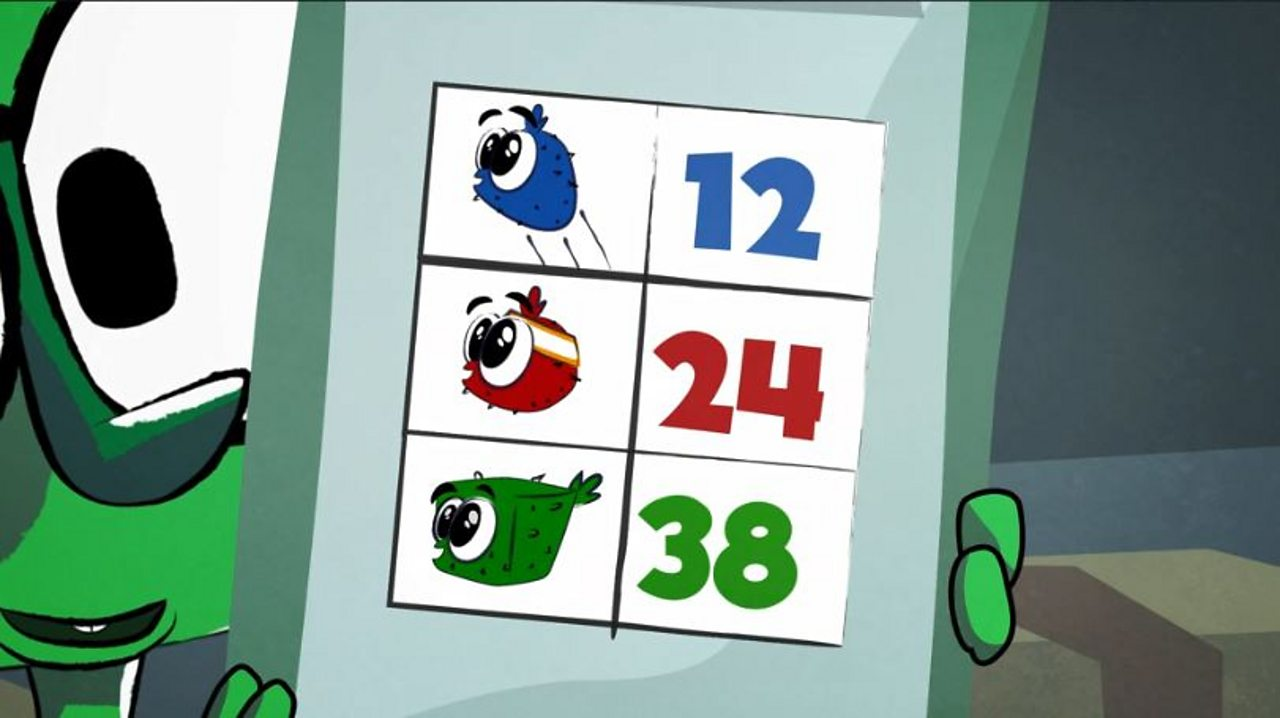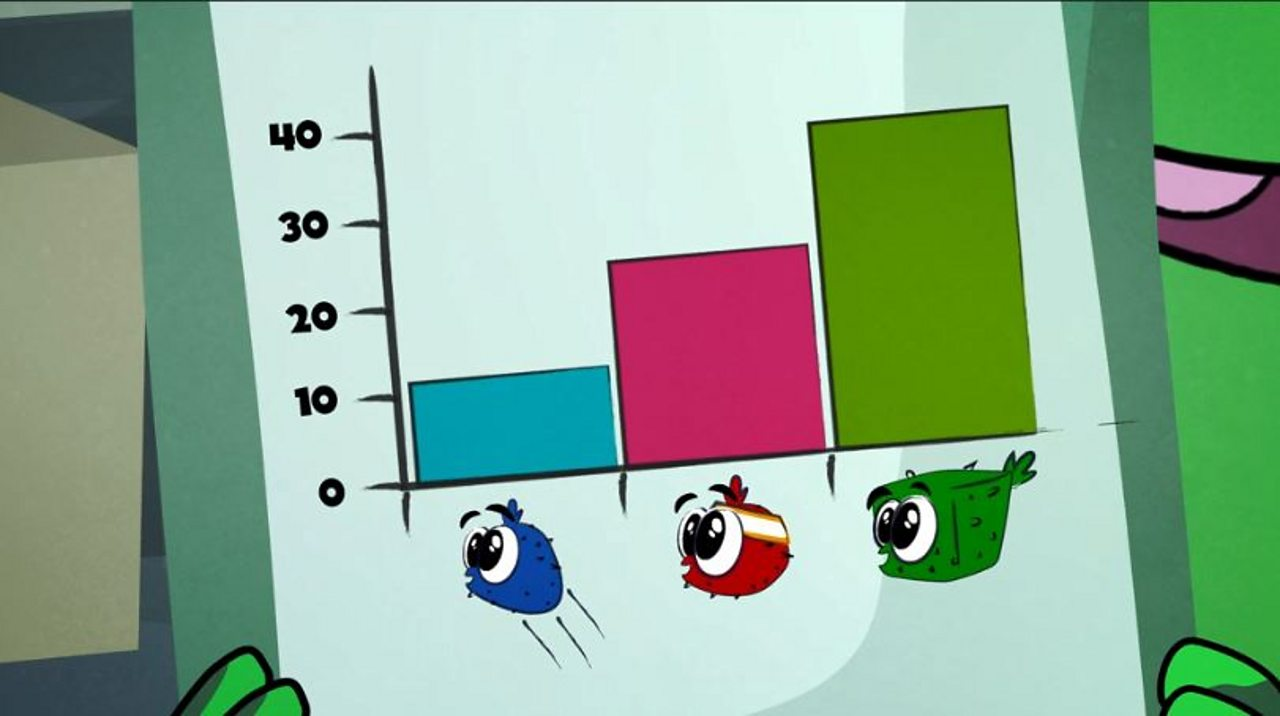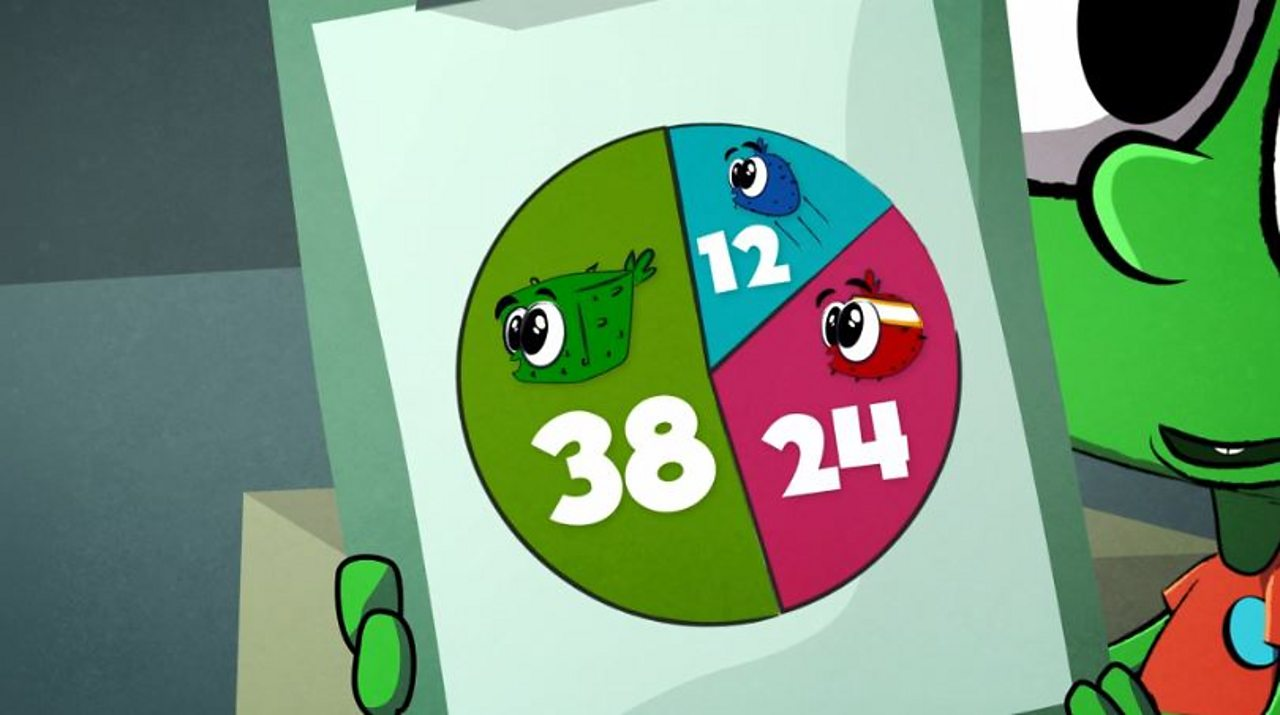How do I record and display data?

How can I count and display data?

A tally is a quick and easy way to record what you are counting.

• Draw a line like a 1 to mark each number you count.
• When you get to 5, draw a diagonal line across the first four lines
• Making groups of 5 like this makes it easier to count your tally later.

How can I show what I have counted?A table shows - what you have counted on the left - how many of each thing there are on the right** as a number.1 of 3A **bar graph** shows: - **what you have counted along the bottom** and **numbers up the left** - the number of each thing is shown by a bar - the **height of the each bar** shows **how many of each thing** there are2 of 3A **pie chart** shows a **total number** as a **circle** - the circle is divided into **different slices for different things** - the **bigger the slice** the **bigger the number** of things3 of 3

How can I show what I have counted?

A table shows:

• what you have counted on the left
• how many of each thing there are on the right as a number

A bar graph shows:

• what you have counted along the bottom and numbers up the left
• the number of each thing is shown by a bar
• the height of the each bar shows how many of each thing there are

A pie chart shows a total number as a circle

• the circle is divided into different slices for different things
• the bigger the slice the bigger the number of things